### 9.5.2.1 Bayesians vs. frequentists

For the past century and a half, there has been a fundamental debate among statisticians on the meaning of probabilities. Virtually everyone is satisfied with the axioms of probability, but beyond this, what is their meaning when making inferences? The two main camps are the frequentists and the Bayesians. A form of Bayes' rule was published in 1763 after the death of Bayes . During most of the nineteenth century Bayesian analysis tended to dominate literature; however, during the twentieth century, the frequentist philosophy became more popular as a more rigorous interpretation of probabilities. In recent years, the credibility of Bayesian methods has been on the rise again.

As seen so far, a Bayesian interprets probabilities as the degree of belief in a hypothesis. Under this philosophy, it is perfectly valid to begin with a prior distribution, gather a few observations, and then make decisions based on the resulting posterior distribution from applying Bayes' rule.

From a frequentist perspective, Bayesian analysis makes far too liberal use of probabilities. The frequentist believes that probabilities are only defined as the quantities obtained in the limit after the number of independent trials tends to infinity. For example, if an unbiased coin is tossed over numerous trials, the probability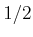represents the value to which the ratio between heads and the total number of trials will converge as the number of trials tends to infinity. On the other hand, a Bayesian might say that the probability that the next trial results in heads is. To a frequentist, this interpretation of probability is too strong.

Frequentists have developed a version of decision theory based on their philosophy; comparisons between the two appear in . As an example, a frequentist would advocate optimizing the following frequentist risk to obtain a decision rule: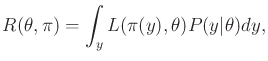(9.88)

in which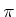represents the strategy,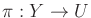. The frequentist risk averages over all data, rather than making a decision based on a single observation, as advocated by Bayesians in (9.26). The probability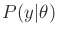is assumed to be obtained in the limit as the number of independent data trials tends to infinity. The main drawback in using (9.88) is that the optimization depends on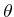. The resulting best decision rule must depend on, which is unknown. In some limited cases, it may be possible to select somethat optimizes (9.88) for all, but this rarely occurs. Thus, the frequentist risk can be viewed as a constraint on the desirability of strategies, but it usually is not powerful enough to select a single one. This problem is reminiscent of Pareto optimality, which was discussed in Section 9.1.1. The frequentist approach attempts to be more conservative and rigorous, with the result being that weaker statements are made regarding decisions.

Steven M LaValle 2020-08-14